# MCAT Physical : Gas Laws

## Example Questions

2 Next →

### Example Question #41 : Fluids And Gases

What is the temperature of a 1L container at STP after the pressure is doubled?

298K

546K

273K

200K

596K

546K

Explanation:

Using Gay-Lussac's Law, which is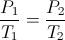, we can find the change in temperature when the pressure is doubled.

Because the problem states that the original conditions were at STP, we know that pressure is 1atm and temperature is 273K. Since pressure and temperature are directly proportional, doubling pressure will also double the temperature. The final temperature will be 546K.

### Example Question #12 : Gas Laws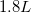of carbon dioxide at an original temperature of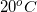is heated to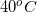. What is the new volume of the gas?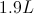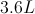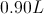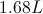Explanation:

Remember to convert temperature to Kelvin when using the gas equations.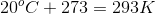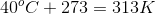We will use Charles's Law to calculate the new volume: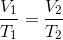Use the given temperatures and initial volume to calculate the final volume.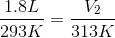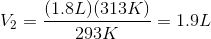### Example Question #42 : Fluids And Gases

Which of the following would not cause a decrease in the pressure of a gas in a sealed container?

Removing gas particles from the container

Reducing the temperature

Adding moles of a different gas

Increasing the volume of the container

Adding moles of a different gas

Explanation:

A decrease in pressure means a decrease in gas particle collisions. The only option that would not cause a decrease in collisions is adding moles of a different gas. Even though different molecules are added, there will be greater pressure as particle collisions will be more frequent.

Reducing temperature slows the gas particles, thus decreasing the frequency of collisions. Similarly, increasing the volume of the container and removing particles will cause a decrease in collisions, and subsequent pressure.

### Example Question #49 : Fluids And Gases

A sealed container holds three moles of gas at 1atm and 200K. Its pressure is to 2atm. What will be the resulting temperature in the container?

400K

300K

100K

250K

273K

400K

Explanation:

In the problem, the volume and the number of moles are constant and the temperature and pressure are the only two variables that are changing. Using the ideal gas law we can find that temperature and pressure are directly proportional. When pressure increases by a factor of two, temperature will also increase by a factor of two.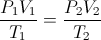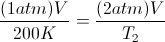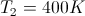### Example Question #11 : Gas Laws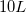container holds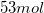of oxygen gas at a temperature of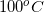. The temperature remains constant and the volume of the container is increased to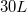. What is the final pressure of the gas in terms of the initial pressure,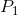?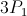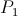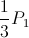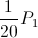Explanation:

The amount of gas is irrelevant. If the temperature is held constant and the volume is increased by a factor of three, the resulting pressure is decreased by a factor of three according to Boyle’s Law.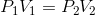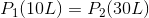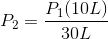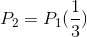### Example Question #13 : Gas Laws

A gas with an initial volume of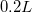and a temperature of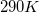is at a pressure of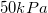. What is the new pressure if the volume is increased to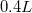and the temperature is increased to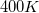?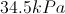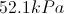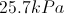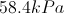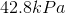Explanation:

To solve this problem we use the combined gas law: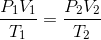Use the given values for the temperature and volume, as well as the initial pressure, to solve for the final pressure.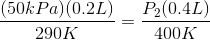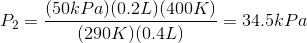2 Next →

### All MCAT Physical Resources